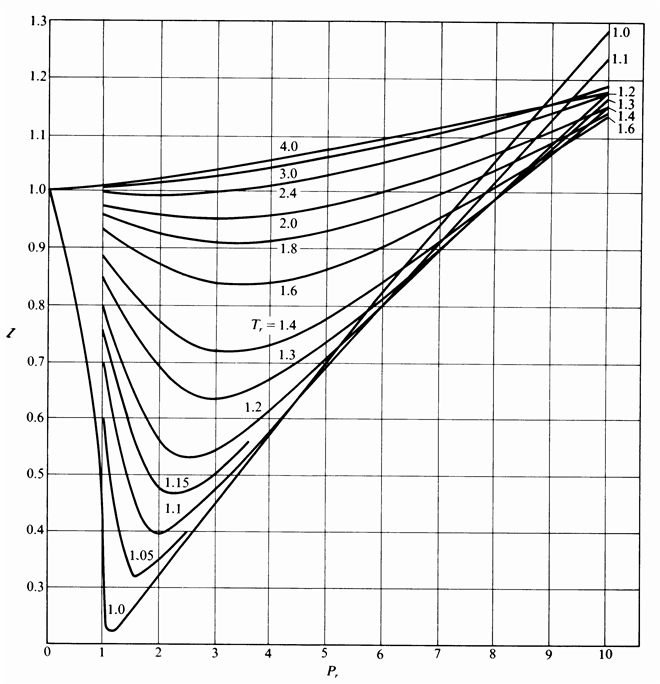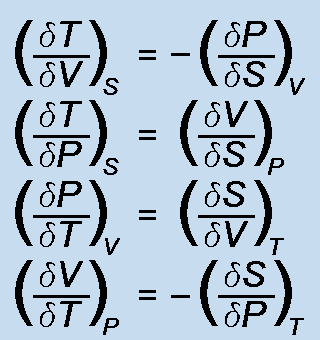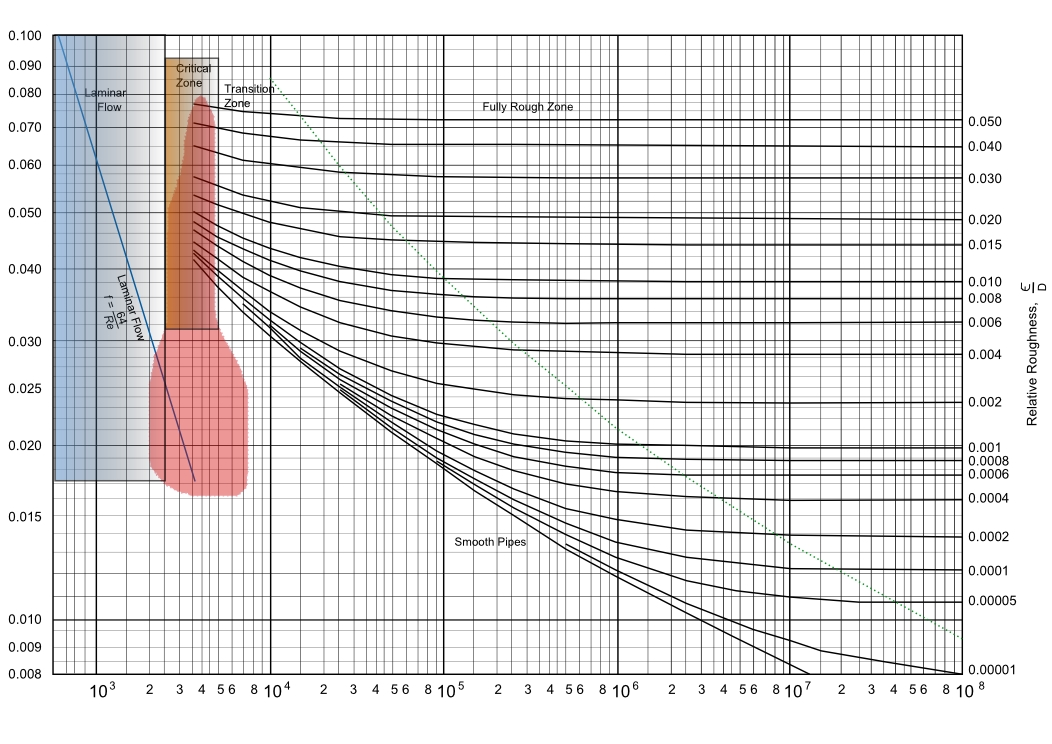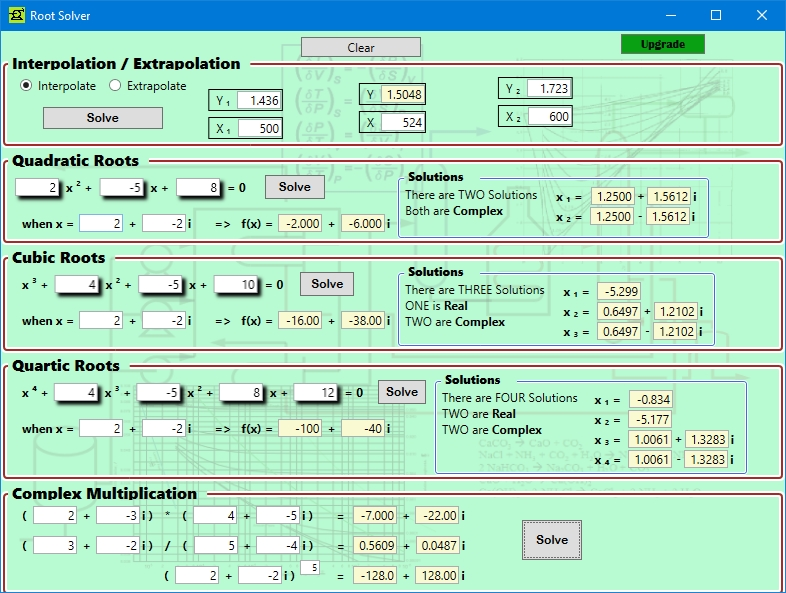### ChemE Soft StoreCaCO3 -> CaO + CO2
NaCl + NH3 + CO2 + H2O -> NaHCO3 + NH4Cl
2 NaHCO3 -> Na2 CO3 + H2O + CO2
CaO + H2 O -> Ca(OH)2
Ca(OH)2 + 2 NH4 Cl -> CaCl2 + 2 NH3 + 2 H2O

## Equation Root Solver

Interpolation/extrapolation. Solve for the roots of quadratic, cubic, and quartic equations, as well as finding the solutions to those functions of x -> y = f(x). Includes complex number math calculations and solutions as well.#### Features:

• Interpolation and extrapolation values are shown relative to each other
• Both real and imaginary roots are calculated
• Functions of any x value can be calculated and shown
• Complex number math includes multiplication, division, and exponential functions